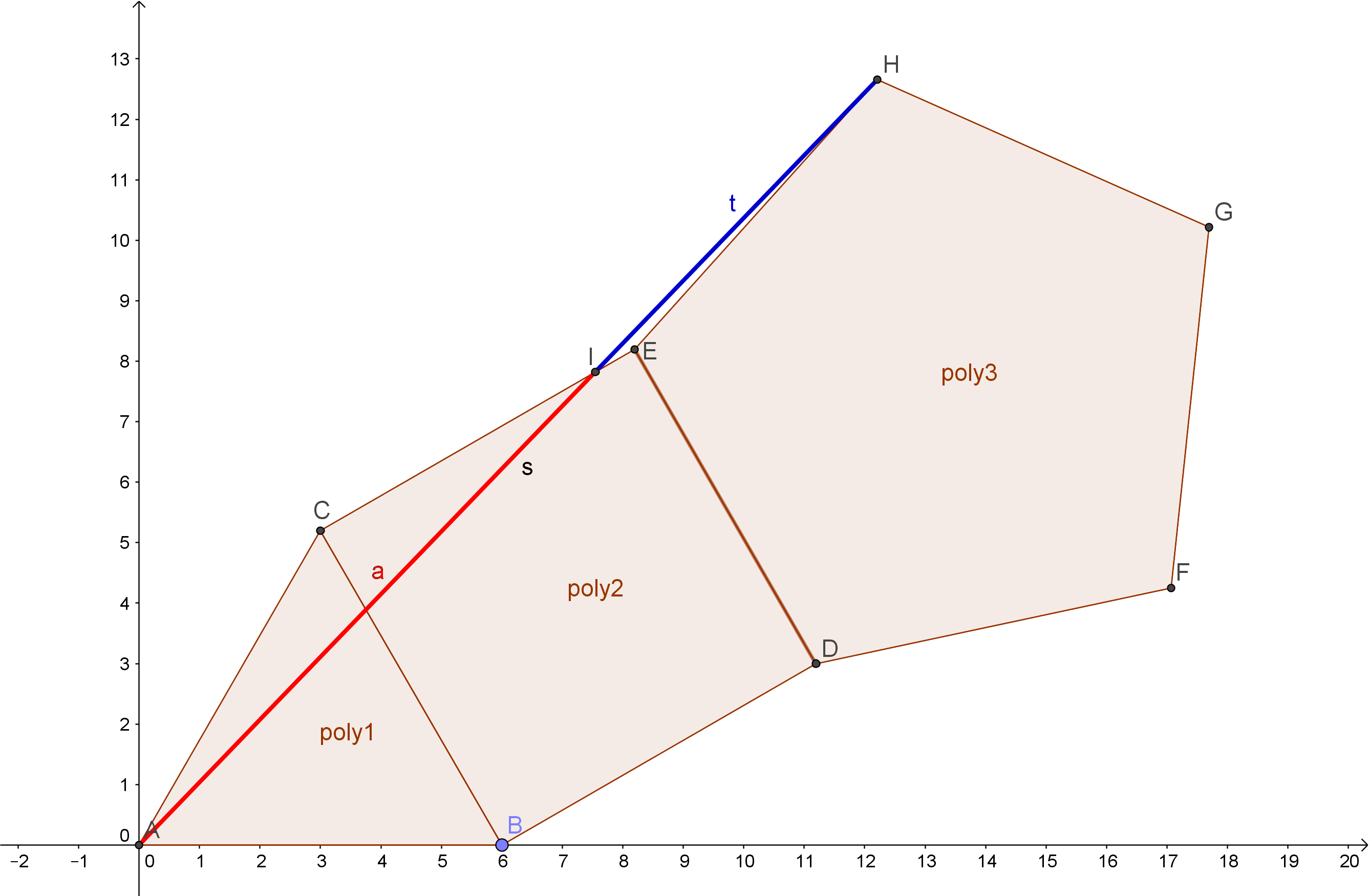# Phi DanceMathematical Surrealism

The sequence starts;
One plus one equals two,
continues; one and two is three;
two and three gives us five…

Phi,
my little friend,
a constant of the approximative value
one comma six one eight!

A ratio
so golden
and a sequence
so pure!

Numbers are juggle balls;
Play around,
my beloved mathemagicians,
and see; It’s all just a game!

Divide one by one,
two by one,
three by two,
five by three,
eight by five
and see:

It will dance around
a mysterious axis,
in this case
called “phi”.

Oscillate,
my little numbers;
dance around the axis
of pure mysteries!

One plus the square root of five
divided by two
creates a number’s hive
of beautiful patterns recognized by you!

View original post

# The Mathemagician’s RoomIsles of Time

It’s a place full of wonder, strangeness and bizarre beauty: the room – or should I say space – of a “mathematician that’s merely a magician.

It’s a common optical illusion, a kind of tricky paradox. “If our brains are simple enough for us to understand them, then we’d be so simple that we couldn’t.” ~ Quote by Ian Steward (a mathematician)

The floor itself is an impossible figure – a typical symbol for paradoxes…
The spirals on the checkerboard-floor are Fibonacci spirals.

This drawing is mostly about the beauty of Phi Φ, Psi ψ and the Fibonacci-sequence.

The fibonacci sequence
The fibonacci sequence works this way:
1+1 = 2
1+2 = 3
2+3 = 5
3+5 = 8
5+8 = 13
8+13 = 21
13+21 = 34
21+34 = 55
34+55 = 89
55+89…
… and so on…
The sequence then is: 1;1;2;3;5;8;13;21;34;55;89;…….

Let’s play with these numbers!
1/1=1
2/1=2
3/2=1.5

View original post 305 more words

# Platonic Solids and the Golden Ratio

There are five regular polyhedra. These are they: tetrahedron, cube/hexahedron, octahedron, dodecahedron and icosahedron. Here is a short summary in form of a table about the vertices, edges, faces and volumes of these solids:

 Tetrahedron Cube/ Hexahedron Octahedron Dodecahedron Icosahedron v vertices 4 8 6 20 12 e edges 6 12 12 30 30 f faces 4 6 8 12 20 V volume √2 /12*a³ a³ √2 /3*a³ (15+7*√5)/4*a³ (15+5*√5)/12*a³

We could group them into three groups:

• The triangular faced ones {tetrahedron, octahedron, icosahedron}

• The square faced one {cube}

• The pentagon faced one {dodecahedron}

If we put these polygons together like this, we can recognize the golden ratio in it: Here we add a triangle, square and a pentagon together in the following way. Then we connect the point A and H with each other – and exactly where this line crosses the square the golden ratio appears:Source
Also, if you didn’t know about the pentagram, even there appears the golden ratio:Source: Wikipedia

And, two of the platonic solids have the golden ratio in themselves: the icosahderon and the dodecahedron.

Imagine 3 planes put together like this:In a dodechedron the exact same planes can be put into it like this:But if we want the edges of the planes to be on the edges of the dodecahedron, we have to use another ratio:Then the ratio is 1 to phi².